Courses

# RS Aggarwal Test: Coordinate Geometry

## 10 Questions MCQ Test Mathematics (Maths) Class 10 | RS Aggarwal Test: Coordinate Geometry

Description
This mock test of RS Aggarwal Test: Coordinate Geometry for Class 10 helps you for every Class 10 entrance exam. This contains 10 Multiple Choice Questions for Class 10 RS Aggarwal Test: Coordinate Geometry (mcq) to study with solutions a complete question bank. The solved questions answers in this RS Aggarwal Test: Coordinate Geometry quiz give you a good mix of easy questions and tough questions. Class 10 students definitely take this RS Aggarwal Test: Coordinate Geometry exercise for a better result in the exam. You can find other RS Aggarwal Test: Coordinate Geometry extra questions, long questions & short questions for Class 10 on EduRev as well by searching above.
QUESTION: 1

Solution:
QUESTION: 2

### The coordinates of the point which is equidistant from the three vertices of the ΔAOB as shown in the figure is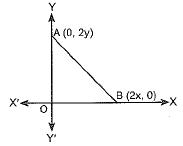Solution:

∵ AOB is a right triangle.
∴ Mid-point of AB is equidistant from A, O and B.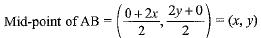QUESTION: 3

### The distance of the point P (2, 3) from the x-axis is

Solution:
QUESTION: 4

If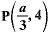is the mid-point of the line segment joining the points Q (-6, 5) and E (-2, 3), then the value of a is

Solution: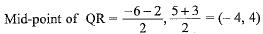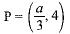So, a/3 = -4 ⇒ a = -12

QUESTION: 5

The distance of the point P (-6, 8) from the origin is

Solution:
QUESTION: 6

The points (k + 1, 1), (2k + 1, 3) and (2k + 2, 2k) are collinear if

Solution:

∵ Points are collinear.
∴ (k + 1) (3 - 2k) + (2k, + 1) (2k - 1) + (2k + 2) (1 - 3) = 0
⇒ 3k+3 - 2k2 - 2k + 4k- 1 - 4k - 4 = 0 ⇒ 2k2 - 3k - 2 = 0
⇒ 2k- 4k + k - 2 = 0
⇒ 2k(k - 2) + 1(k - 2) = 0
⇒ (2k + 1) (k - 2) = 0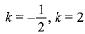QUESTION: 7

The point A (-5, 6) is at a distance of

Solution:
QUESTION: 8

The coordinates of the point which is equidistant from the three vertices of the triangle shown in the given Fig. 6.6 are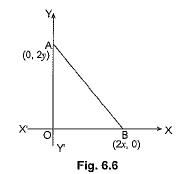Solution:
QUESTION: 9

The area of a triangle with vertices A (3, 0), B (7, 0) and C (8, 4) is

Solution:
QUESTION: 10

The points which lies on the perpendicular bisector of the line segment joining the points A (-2, -5), B (2, 5) is

Solution: